# 2003COMC加拿大数学公开赛真题答案免费下载

### 真题与答案下载### 2003 COMC真题答案免费下载

Part A共8题，每题5分

Part B共4题，每题10分

#### 部分真题预览：

Part A Solutions:

A3)The particle which moves clockwise is moving three times as fast as the particle moving counterclockwise. Therefore, the particle moving clockwise moves three times as far as the particle moving counterclockwise in the same amount of time.
This tells us that in the time that the clockwise particle travels 3/4 of the way around the circle, the counterclockwise particle will travel 1/4 of the way around the circle, and so the two particles will meet at P(0,1) .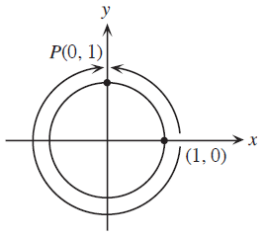Using the same reasoning, the particles will meet at Q(−1, 0) when they meet the second time.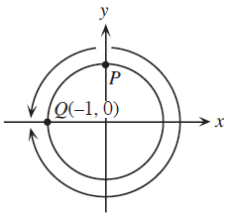Part B Solutions:

B2)

1. Two Solutions:
1. Solution 1:Drop perpendiculars from D and A to BC, meeting BC at E and F respectively.
Since DA is parallel to CB, then DE and AF are also perpendicular to DA.
Since DAFE is a rectangle, then EF = 6.
Since DC = AB, DE = AF and ΔDEC and ΔAFB are rightangled, then they are congruent triangles, and so CE = BF , and so both of these lengths must be equal to 3.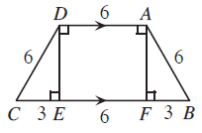By the Pythagorean Theorem, DE = √(DC2 − CE2) =√( 62 − 32 )= √27 =3√3.
Therefore, the sides of ΔDEC are in the ratio 1 to √3 to 2, so DEC is a 30-60-90 triangle, with∠ DCE = 60° and ∠ CDE = 30° .
Therefore, since we have congruent triangles, ∠ DCB = ∠ABC = ∠DCE = 60° and ∠ CDA = ∠DAB = 90° +∠CDE =120° .
1. If Chuck was attached to a point P and there were no obstructions, he would be able to reach a circle of radius 8 m. (If Chuck stays at the end of his chain, he could trace out a circle of radius 8 m, but Chuck can move everywhere inside this circle, since his chain does not have to be tight.) However, here we have an obstruction – the trapezoidal barn. Since the interior angle of the barn at point A is 120° , then the exterior angle of the barn is 240° . So Chuck can certainly reach the area which is a 240° sector of radius 8 m, centred at A. (If Chuck extends the chain as far as possible in a straight line in the direction of D from A, can then walk in a clockwise direction, keeping the chain at its full length until the chain lies along AB. He will have moved through 240° , and the region is the sector of a circle.)
However, when the chain is fully extended in the direction of D, Chuck will be 2 m past point D. He will thus be free to move towards side DC of the barn. If he does this and keeps the chain tight, he will trace out part of a circle of radius 2 m centred at D. (Point D now serves as a “pivot” point for the chain.) Since the exterior angle of the barn at point D is 240° , then the angle between AD extended and DC is 60° . Therefore, Chuck can reach a 60° sector of a circle of radius 2 m, centred at D.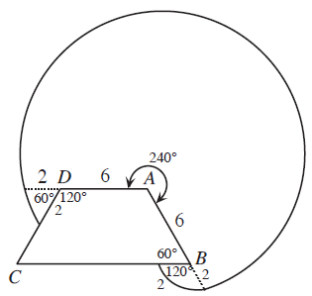When the chain is fully extended in the direction of B, Chuck will be 2 m past point B. He will thus be free to move towards side BC of the barn. If he does this and keeps the chain tight, he will trace out part of a circle of radius 2 m centred at B. (Point B now serves as a “pivot” point for the chain.) Since the exterior angle of the barn at point B is 300° (the interior angle at B is 60° ), then the angle between AB extended and BC is 120° . Therefore, Chuck can reach a 120° sector of a circle of radius 2 m, centred at B.
The area of a sector of angle θ o of a circle of radius r is (θ/360)πr2 .
Therefore, the total area that Chuck can reach is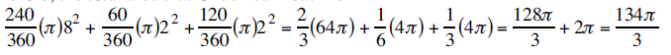square metres.

### 2003 COMC加拿大数学奥赛完整版真题免费下载